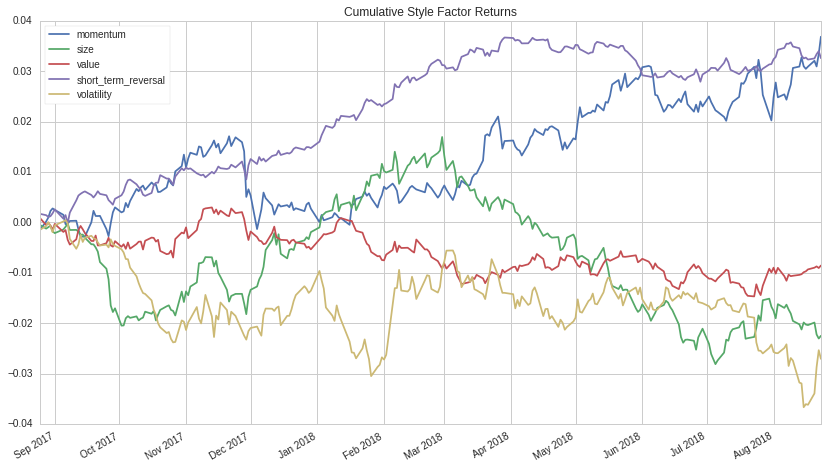Notebook

Common Risk Factor Performance¶

by Max Margenot

This is a quick notebook meant to provide a snapshot of the performance of common risk factors over the past year, using whatever today is as a reference point.

This notebook is more useful for getting a sense of the behavior of the market over the past year than it is for algorithm development, but it's always important to keep in mind that algorithms run within the context of the market. As market regimes shift, the way your strategy behaves may change, so keeping an eye on the market should prove useful, even if you are designing a strategy with neutrality to common factor risk in mind.

For more information on the common risk factors used in this notebook, check out the Quantopian Risk Model.

In :
from quantopian.research.experimental import get_factor_returns
import matplotlib.pyplot as plt
import datetime as dt
import qgrid

In :
today = dt.datetime.today() - dt.timedelta(days=1)
last_year = dt.datetime.today() - dt.timedelta(weeks=52)

In :
factor_returns = get_factor_returns(start=last_year, end=today)


Here is a quick use-case of qgrid. Try examining the performance of style risk factors when you filter the sector risk factor returns to within $r_{factor} &lt; |0.2|$, or try examining how momentum performs on days where size is small.

In :
qgrid_widget = qgrid.show_grid(factor_returns, show_toolbar=False)

In :
qgrid_widget


Failed to display Jupyter Widget of type QgridWidget.

If you're reading this message in the Jupyter Notebook or JupyterLab Notebook, it may mean that the widgets JavaScript is still loading. If this message persists, it likely means that the widgets JavaScript library is either not installed or not enabled. See the Jupyter Widgets Documentation for setup instructions.

If you're reading this message in another frontend (for example, a static rendering on GitHub or NBViewer), it may mean that your frontend doesn't currently support widgets.

Summary Statistics of Common Risk Factor Returns¶

Here we calculate the summary statistics of sector and style risk factors over the past year.

In :
summary_stats = factor_returns.describe()

In :
summary_stats[[
'basic_materials',
'consumer_cyclical',
'financial_services',
'real_estate',
'consumer_defensive',
'health_care',
'utilities',
'communication_services',
'energy',
'industrials',
'technology'
]]

Out:
basic_materials consumer_cyclical financial_services real_estate consumer_defensive health_care utilities communication_services energy industrials technology
count 251.000000 251.000000 251.000000 251.000000 251.000000 251.000000 251.000000 251.000000 251.000000 251.000000 251.000000
mean 0.000411 0.001106 0.000638 0.000257 0.000109 0.000682 0.000062 -0.000134 0.000856 0.000626 0.001076
std 0.009502 0.008381 0.010480 0.007528 0.007683 0.008640 0.008217 0.010126 0.011180 0.009286 0.010509
min -0.035444 -0.039686 -0.050247 -0.029336 -0.036302 -0.044254 -0.031027 -0.036232 -0.042230 -0.045268 -0.041811
25% -0.004431 -0.003031 -0.003718 -0.003888 -0.003582 -0.002895 -0.005085 -0.006074 -0.004294 -0.003075 -0.003232
50% 0.000682 0.001790 0.000691 0.000500 0.000185 0.001023 0.000355 0.000359 0.001299 0.001402 0.001198
75% 0.006140 0.005283 0.006125 0.005290 0.004546 0.005801 0.005160 0.005582 0.007260 0.006005 0.006302
max 0.027198 0.029550 0.033010 0.022006 0.016333 0.021426 0.026289 0.035204 0.033102 0.023492 0.038438
In :
summary_stats[[
'momentum',
'size',
'value',
'short_term_reversal',
'volatility'
]]

Out:
momentum size value short_term_reversal volatility
count 251.000000 251.000000 251.000000 251.000000 251.000000
mean 0.000146 -0.000089 -0.000034 0.000128 -0.000107
std 0.001710 0.001638 0.001047 0.000811 0.001988
min -0.008917 -0.005244 -0.002409 -0.001921 -0.005018
25% -0.000918 -0.001098 -0.000771 -0.000463 -0.001329
50% 0.000245 0.000116 -0.000071 0.000120 -0.000186
75% 0.001313 0.000898 0.000617 0.000598 0.001122
max 0.004884 0.004830 0.003704 0.002883 0.010091

Performance Statistics¶

Here we plot the mean return of each common risk factor and the volatility of the returns of each risk factor. It seems like the volatility of each style factor is generally lower than the volatility of each sector factor, though more observations should be accumulated before stating that with confidence.

In :
summary_stats.loc['mean'].plot(kind='bar',
title='Mean of Common Risk Factor Returns');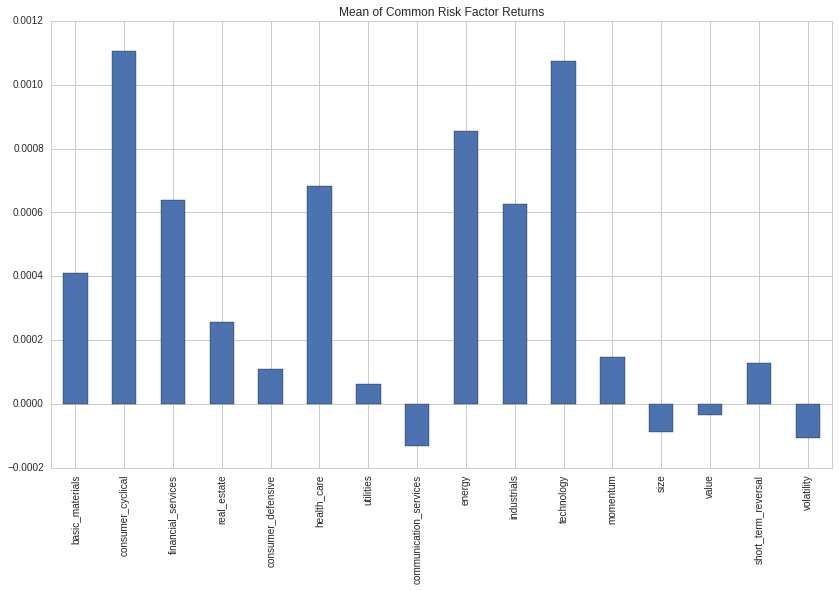In :
summary_stats.loc['std'].plot(kind='bar',
title='Volatility of Common Risk Factor Returns');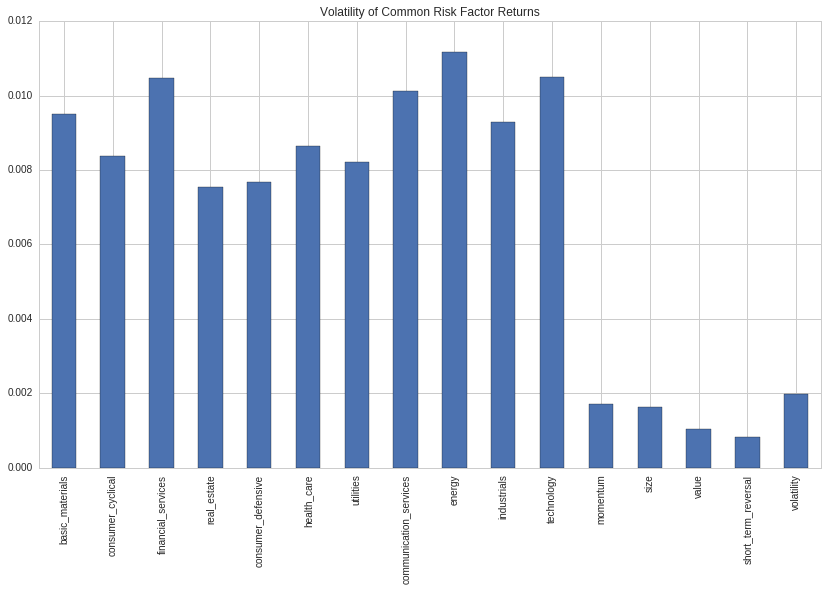Daily Common Factor Returns¶

Choose which risk factor you'd like to examine in more detail. The following plot will show it alongside bars for one, two, and three standard deviations away from the mean return. The idea here is to get a sense of the outliers in the returns of risk factors over the past year.

In :
selected_risk_factor = 'momentum'

In :
factor_returns[selected_risk_factor].plot(title='{0} Risk Factor Returns'.format(selected_risk_factor))
# First standard deviation
plt.axhline(summary_stats[selected_risk_factor].loc['mean'] + \
summary_stats[selected_risk_factor].loc['std'],
linestyle='--',
color='purple',
alpha=1.0,
label='1 Standard Deviation of {0} Returns'.format(selected_risk_factor))
plt.axhline(summary_stats[selected_risk_factor].loc['mean'] - \
summary_stats[selected_risk_factor].loc['std'], linestyle='--', color='purple', alpha=1.0)
# Second standard deviation
plt.axhline(summary_stats[selected_risk_factor].loc['mean'] + \
2 * summary_stats[selected_risk_factor].loc['std'],
linestyle='--',
color='orange',
alpha=1.0,
label='2 Standard Deviations of {0} Returns'.format(selected_risk_factor))
plt.axhline(summary_stats[selected_risk_factor].loc['mean'] - \
2 * summary_stats[selected_risk_factor].loc['std'], linestyle='--', color='orange', alpha=1.0)
# Third standard deviation
plt.axhline(summary_stats[selected_risk_factor].loc['mean'] + \
3 * summary_stats[selected_risk_factor].loc['std'],
linestyle='--',
color='r',
alpha=1.0,
label='3 Standard Deviations of {0} Returns'.format(selected_risk_factor))
plt.axhline(summary_stats[selected_risk_factor].loc['mean'] - \
3 * summary_stats[selected_risk_factor].loc['std'], linestyle='--', color='r', alpha=1.0)
plt.legend();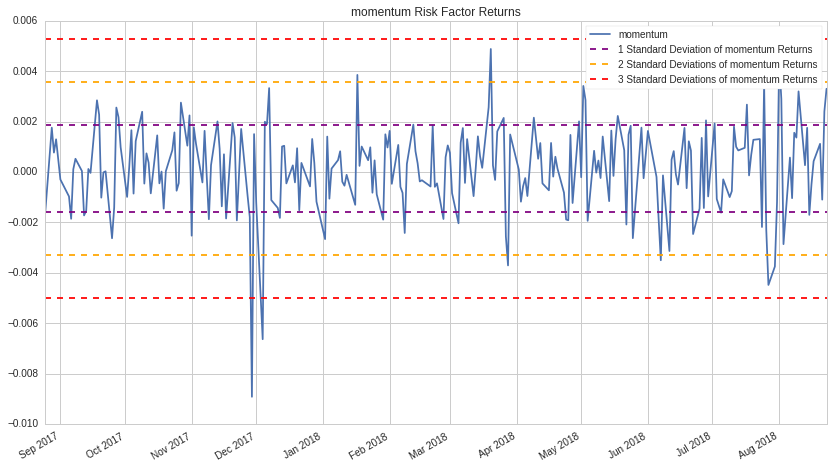Cumulative Returns of Common Risk Factors¶

And, finally, here we examine the cumulative returns of each common risk factor. Sector factors seem to be collectively impacted by significant market shifts, while style factors each react differently.

In :
((1 + factor_returns[[
'basic_materials',
'consumer_cyclical',
'financial_services',
'real_estate',
'consumer_defensive',
'health_care',
'utilities',
'communication_services',
'energy',
'industrials',
'technology'
]]).cumprod() - 1).plot(title='Cumulative Sector Factor Returns');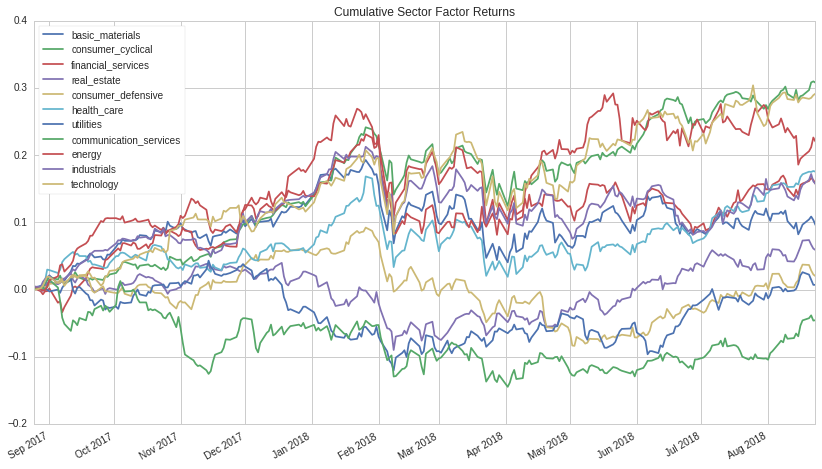In :
((1 + factor_returns[[
'momentum',
'size',
'value',
'short_term_reversal',
'volatility'
]]).cumprod() - 1).plot(title='Cumulative Style Factor Returns');